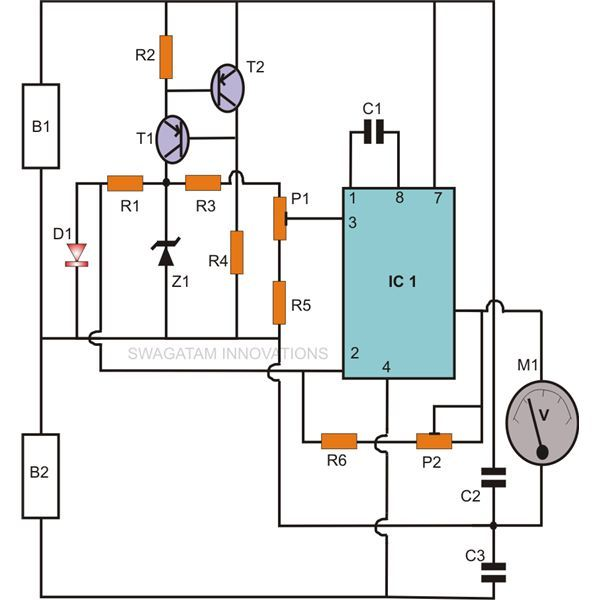Home » Electronic Sensors » Room Temperature Monitor Circuit# Room Temperature Monitor Circuit

A very simple yet highly precise air temperature sensor gauge circuit has been presented here.

The use of the highly versatile and accurate IC LM 308 makes the circuit respond and react superbly to the smallest temperature changes happening over its surrounding atmosphere.

## Using the Garden Diode 1N4148 as the Temperature Sensor

Diode 1N4148 (D1) is used as an active ambient temperature sensor here. The unique drawback of a semiconductor diode such as a 1N4148 which shows forward voltage characteristic change with the influence of ambient temperature change has been effectively exploited here, and this device is used as a efficient, cheap temperature sensor.

The electronic air temperature sensor gauge circuit presented here is very accurate in its function, categorically due to its minimum level of hysteresis.

Complete circuit description and construction clues included herein.

## Circuit Operation

The present circuit of an electronic air temperature sensor gauge circuit is outstandingly accurate and can be very effectively used to monitor the atmospheric temperature variations. Let’s briefly study its circuit functioning:

Here as usual we use the very versatile “garden diode” 1N4148 as the sensor due to its typical drawback (or rather an advantage for the present case) of changing its conduction characteristic in the influence of a varying ambient temperature.

The diode 1N4148 is comfortably able to produce a linear and an exponential voltage drop across itself in response to a corresponding increase in the ambient temperature.

This voltage drop is around 2mV for every degree rise in temperature.

This particular feature of 1N4148 is extensively exploited in many low range temperature sensor circuits.

Referring to the proposed room temperature monitor with indicator circuit diagram given below, we see that, IC1 is wired as an inverting amplifier and forms the heart of the circuit.

Its non inverting pin # 3 is held at a particular fixed reference voltage with the help of Z1, R4, P1 and R6.

Transistor T1 and T2 are used as a constant current source and helps in maintaining higher accuracy of the circuit.

The inverting input of the IC is connected to the sensor and monitors even the slightest change in the voltage variation across the sensor diode D1. These voltage variations as explained, is directly proportional to the changes in the ambient temperature.

The sensed temperature variation is instantly amplified into a corresponding voltage level by the IC and is received at its output pin #6.

The relevant readings are directly translated into degree Celsius through a 0-1V FSD moving coil type meter.

Parts List

R1, R4 = 12K,
R2 = 100E,
R3 = 1M,
R5 = 91K,
R6 = 510K,
P1 = 10K PRESET,
P2 = 100K PRESET,
C1 = 33pF,
C2, C3 = 0.0033uF,
T1, T2 = BC 557,
Z1= 4.7V, 400mW,
D1 =1N4148,
IC1 = LM308,
General Purpose Board as per size.
B1 and B2 = 9V PP3 battery.
M1 = 0 – 1 V, FSD moving coil type voltmeter

#### Setting Up the Circuit

The procedure is a bit critical and requires special attention. To complete the procedure you will need two accurately known temperature sources (hot and cold) and an accurate mercury-in-glass thermometer.

The calibration may be completed through the following points:

Initially keep the presets set at their midways. Connect a voltmeter (1 V FSD) at the output of the circuit.

For the cold temperature source, water at about room temperature is used here.

Dip the sensor and the glass thermometer into the water and record the temperature in the glass thermometer and the equivalent voltage outcome in the voltmeter.

Take a bowl of oil, heat it to about 100 degrees Celsius and wait until its temperature stabilizes down to about 80 degrees Celsius.

As above, immerse the two sensors and compare them with the above result. The voltage reading should be equal to the temperature change in the glass thermometer times 10 mill volt. Didn’t get it? Well, let’s read the following example.

Suppose, the cold temperature source water is at 25 degrees Celsius (room temperature), the hot source, as we know is at 80 degrees Celsius. Thus, the difference or the temperature change between them is equal to 55 degrees Celsius. Therefore the difference in the voltage readings should be 55 multiplied by 10 = 550 mill volts, or 0.55 volts.

If you don’t quite get the criterion satisfied, adjust P2 and continue to repeat the steps, until finally you achieve it.
Once the above rate of change (10 mV per 1 degree Celsius) is set, just adjust P1 so that the meter shows 0.25 volts at 25 degrees (sensor held in water at room temperature).

This concludes the setting of the circuit.
This air temperature sensor gauge circuit can also be effectively used as an electronic room thermometer unit.

SHARING IS CARING!### About the Author

I am an electronic engineer (dipIETE ), hobbyist, inventor, schematic/PCB designer, manufacturer. I am also the founder of the website: https://www.homemade-circuits.com/, where I love sharing my innovative circuit ideas and tutorials. If you have any circuit related query, you may interact through comments, I'll be most happy to help!